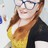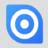Order reference field (static text, last 2 digits of date, auto number)Hi again

I'd like to give orders a reference number structured like:

OR_19_001Accidentally submitted!

Anyway, above structure where

OR = statis text

19 = last 2 digits of the order date (i.e the year)

001 = auto-increasing number

Having trouble wrapping my head around the best way to do so.Hi Sarah,

you can use the following formula for that in the option "Trigger after create" in the table properties or in a button.

`let myyear := format(today(), "YY");`
`let myOrderNr := max((select Purchases where substr('Order number', 3, 2) = myyear).number(substr('Order number', 6)));`
`if cnt(myOrderNr) < 1 then`
`'Order number' := text("OR_" + number(format(today(), "YY")) + "_0001")`
`else`
`'Order number' := text("OR_" + number(format(today(), "YY")) + "_" + format(myOrderNr + 1, "0000"))`
`end`

Kind regards, Jörg